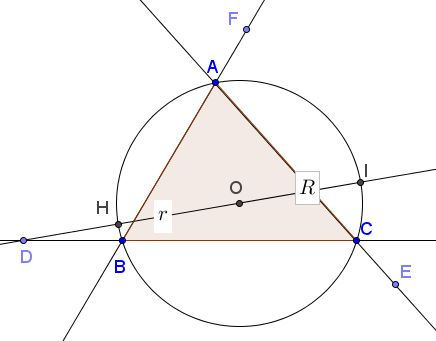# Two Triangles Sharing the Circumcenter

In $\Delta ABC$ points $D,\,E,\,F$ lie on sides $BC,\,AC,\,AB,$ respectively, satisfy

$\displaystyle\frac{BD}{DC}=\frac{CE}{EA}=\frac{AF}{FB}.$

Points $D,\,E,\,F$ are either all strictly internal or all strictly external to the sides of $\Delta ABC.$ Prove that, if triangles $ABC$ and $DEF$ share the circumcenter, they are both equilateral.

### Proof 1

Assume points $D,\,E,\,F$ are internal to the sides of $\Delta ABC.$ Set $R$ the circumradius of $\Delta ABC,$ $r$ that of $\Delta DEF,$ $k^{2}$ the common ratio above, $O$ the common circumcenter.With the reference to the above diagram, the power of point $D$ relative to the circumcircle $(ABC)$ is

$B\cdot DC=AH\cdot D=(R-r)(R+r)=R^{2}-r^{2}.$

Similarly, $AC\cdot EA=A\cdot FB=R^{2}-r^{2}.$ Denoting $p^{2}=R^{2}-r^{2},$ we then have, for example, $B\cdot DC=p^{2}$ and $\displaystyle\frac{BD}{DC}=k^{2},$ from which $B=k,$ $\displaystyle DC=\frac{p}{k}.$ By the same token, $AC=A=k,$ $\displaystyle EA=FB=\frac{p}{k}.$

It thus follows that $B=AC=A$ and $DC=EA=FB,$ from which

$BC=BD+DC=CE+EA=AC,$

and, similarly, $AC=AB,$ making triangle $ABC$ equilateral. Triangle $DEF$ now is obtained from $\Delta ABC$ by a spiral similarity and is, therefore, also equilateral.

In case points $D,\,E,\,F$ are located on the extensions of the sides of $\Delta ABC,$ we'll have to define $p^{2}=r^{2}-R^{2},$ leaving the rest unchanged.### Proof 2

Identify the given points with complex numbers so that $A=1,$ $B=e^{it},$ $C=e^{iu},$ with $0\lt t\lt u\lt 2\pi.$ Denote the common ratio as $k;$ this is a real number different from $0$ or $1.$ We have,

$\displaystyle D=\frac{e^{it}+ke^{iu}}{1+k},$ $\displaystyle E=\frac{e^{iu}+k}{1+k},$ $\displaystyle F=\frac{ke^{it}+1}{1+k}.$

But $|e^{it}+ke^{iu}|=|e^{iu}+k|=|1+ke^{it}|,$ implying

$\cos (u-t)=\cos u=\cos t.$

From $\cos u=\cos t$ we have either $u=t$ or $u+t=2\pi.$ In the former case we would have $B=C,$ which has been excluded. Thus one case remains: $u+t=2\pi.$

From $\cos (u-t)=\cos t$ we have either $u-t=t$ or $u=2\pi.$ The latter is impossible. Thus one case remains: $u=2t,$ implying $3t=2\pi,$ i.e., $\displaystyle t=\frac{2\pi}{3},$ $\displaystyle u=\frac{4\pi}{3}.$ So $\Delta ABC$ is indeed equilateral.

### Acknowledgment

The problem has been posted by Leo Giugiuc at CutTheKnotMath along with his two solutions.